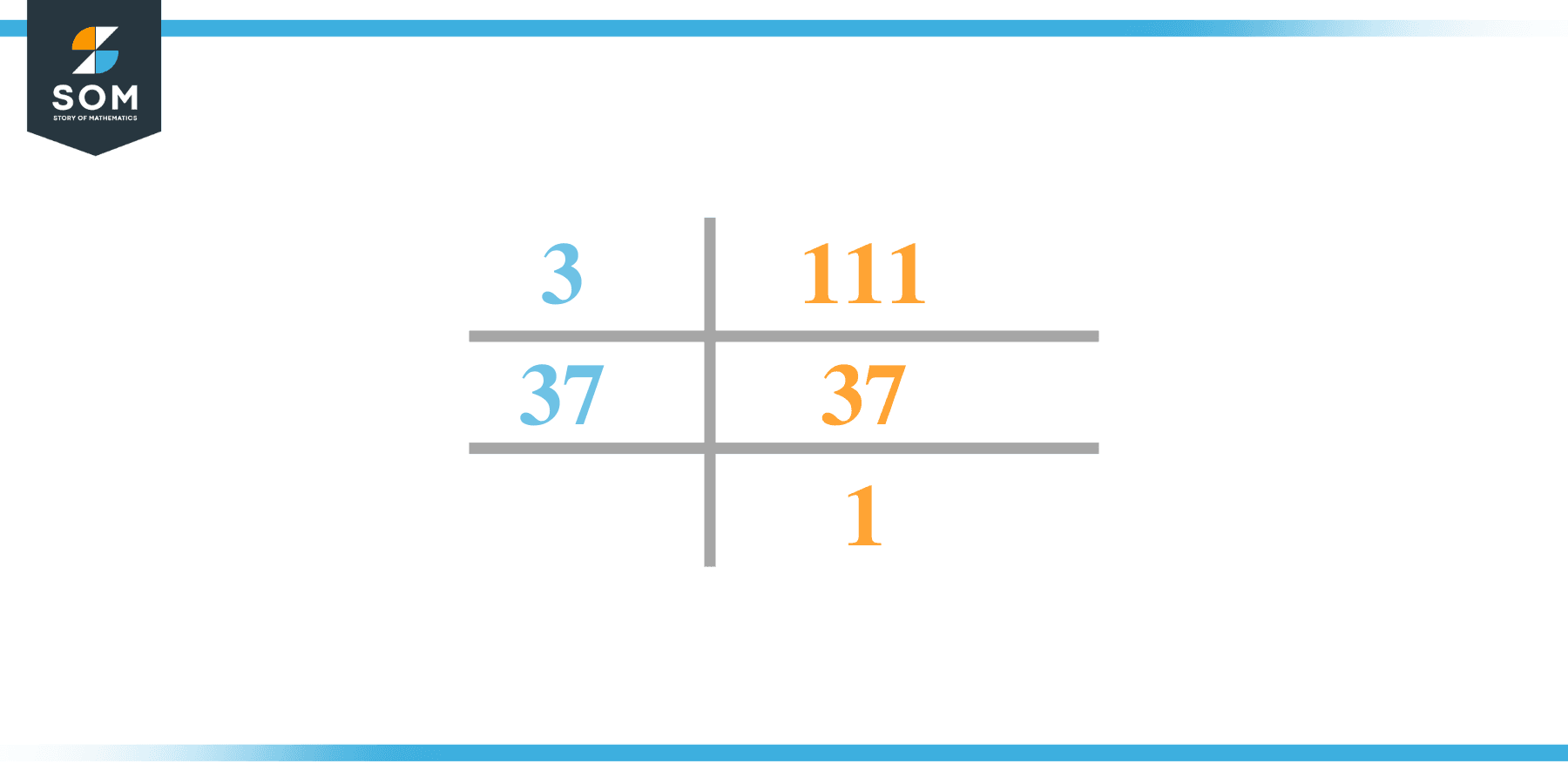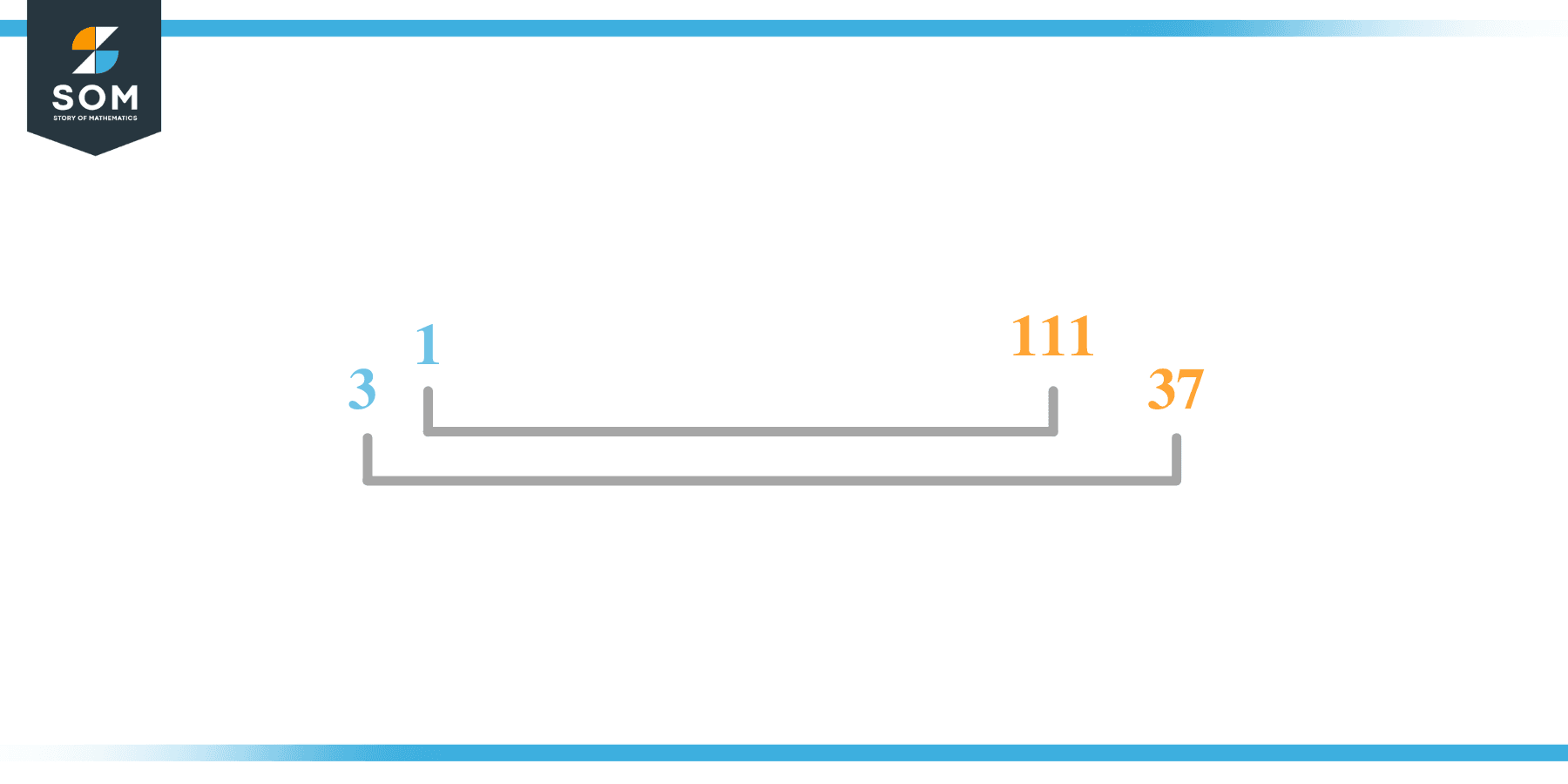# Factors of 111: Prime Factorization, Methods, and Examples

A number can be divided equally into its constituents called factors. Factors can also be defined as whole numbers that divide a particular number wholly. 111 is called a composite number because it has more than two factors.### Factors of 111

Here are the factors of number 111.

Factors of 111: 1, 3, 37, 111

### Negative Factors of 111

The negative factors of 111 are similar to its positive factors, just with a negative sign.

Negative Factors of 111: -1, -3, -37 and -111

### Prime Factorization of 111

The prime factorization of 111 is the way of expressing its prime factors in the form of the product.

Prime Factorization: 3 x 37

In this article, we will learn about the factors of 111 and how to find them using various techniques such as upside-down division, prime factorization, and factor tree.

## What Are the Factors of 111?

The factors of 111 are 1, 3, 37, and 111. All of these numbers are the factors as they do not leave any remainder when divided by 111.

The factors of 111 are classified as prime numbers and composite numbers. The prime factors of the number 111 can be determined using the technique of prime factorization.

## How To Find the Factors of 111?

You can find the factors of 111 by using the rules of divisibility. The rule of divisibility states that any number when divided by any other natural number then it is said to be divisible by the number if the quotient is the whole number and the resulting remainder is zero.

To find the factors of 111, create a list containing the numbers that are exactly divisible by 111 with zero remainders. One important thing to note is that 1 and 111 are 111’s factors as every natural number has 1 and the number itself as its factor.

1 is also called the universal factor of every number. The factors of 111 are determined as follows:

$\dfrac{111}{1} = 111$

$\dfrac{111}{3} = 37$

$\dfrac{111}{37} = 3$

$\dfrac{111}{111} = 1$

Therefore 1, 3, 37, and 111 are the factors of 111.

### Total Number of Factors of 111

For 111 there are 4 positive factors and 4 negative ones. So in total, there are 8 factors of 111.

To find the total number of factors of the given number, follow the procedure mentioned below:

1. Find the factorization/prime factorization of the given number.
2. Demonstrate the prime factorization of the number in the form of exponent form.
3. Add 1 to each of the exponents of the prime factor.
4. Now, multiply the resulting exponents together. This obtained product is equivalent to the total number of factors of the given number.

By following this procedure the total number of factors of 111 is given as:

The factorization of 111 is 1x 3 x 37.

The exponent of 1, 3, and 37 is.

Adding 1 to each and multiplying them together results in 8.

Therefore, the total number of factors of 111 is 8. 4 are positive and 4 factors are negative.

### Important Notes

Here are some important points that must be considered while finding the factors of any given number:

• The factor of any given number must be a whole number.
• The factors of the number cannot be in the form of decimals or fractions.
• Factors can be positive as well as negative.
• Negative factors are the additive inverse of the positive factors of a given number.
• The factor of a number cannot be greater than that number.
• Every even number has 2 as its prime factor which is the smallest prime factor.

## Factors of 111 by Prime FactorizationThe number 111 is a composite. Prime factorization is a useful technique for finding the number’s prime factors and expressing the number as the product of its prime factors.

Before finding the factors of 111 using prime factorization, let us find out what prime factors are. Prime factors are the factors of any given number that are only divisible by 1 and themselves.

To start the prime factorization of 111, start dividing by its smallest prime factor. First, determine that the given number is either even or odd. If it is an even number, then 2 will be the smallest prime factor.

Continue splitting the quotient obtained until 1 is received as the quotient. The prime factorization of 111 can be expressed as:

111 = 3 x 37

## Factors of 111 in PairsThe factor pairs are the duplet of numbers that when multiplied together result in the factorized number. Depending upon the total number of factors of the given numbers, factor pairs can be more than one.

For 111, the factor pairs can be found as:

1 x 111 = 111

3 x 37 = 111

The possible factor pairs of 111 are given as (1, 111) and(3, 37 ).

All these numbers in pairs, when multiplied, give 111 as the product.

The negative factor pairs of 11 are given as:

-1 x -111 = 111

-3 x -37 = 111

It is important to note that in negative factor pairs, the minus sign has been multiplied by the minus sign due to which the resulting product is the original positive number. Therefore, -1, -3, -37, and -111 are called negative factors of X.

The list of all the factors of 111 including positive as well as negative numbers is given below.

Factor list of 111: 1, -1, 3, -3, 37, -37, 111, and -111

## Factors of 111 Solved Examples

To better understand the concept of factors, let’s solve some examples.

### Example 1

How many factors of 111 are there?

### Solution

The total number of Factors of 111 is 4.

Factors of 111 are 1, 3, 37, and 111.

### Example 2

Find the factors of 111 using prime factorization.

### Solution

The prime factorization of 111 is given as:

111 $\div$ 3 = 37

37 \$\div4 37 = 1

So the prime factorization of 111 can be written as:

3 x 37 = 111